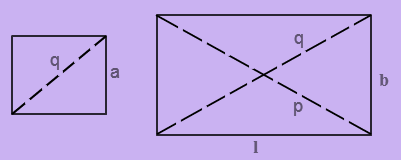# Diagonal Formula

The line stretching from one corner of the square or rectangle to the opposite corner through the centre of the figure is known as the diagonal. Any square that has two diagonals are equal in length to each other. Diagonal Formula is used to calculate the polygon diagonals. Diagonals are a line joining two nonadjacent vertices of a polygon i.e. a diagonal joins two vertices of a polygon excluding the edges of the figure.### Diagonal of a Square

$$\begin{array}{l}Diagonal\ of \ square=a\sqrt{2}\end{array}$$

Where, a is the length of the side of the square

### Diagonal of a Rectangle

$$\begin{array}{l}\ Diagonal\;of\;a\;Rectangle=\sqrt{l^{2}+b^{2}}\end{array}$$

Where,
l is the length of the rectangle.
b is the breadth of the rectangle.
p and q are the diagonals

### Diagonal of a Parallelogram

Formula of parallelogram diagonal in terms of sides and cosine

$$\begin{array}{l}\beta\end{array}$$
(cosine theorem)

$$\begin{array}{l}\ p=d_{1}=\sqrt{a^{2}+b^{2}- 2ab\;cos \beta}\end{array}$$

$$\begin{array}{l}q=d_{2}=\sqrt{a^{2}+b^{2}+ 2ab\; cos \beta}\end{array}$$

Formula of parallelogram diagonal in terms of sides and cosine α (cosine theorem)

$$\begin{array}{l}\ p=d_{1}=\sqrt{a^{2}+b^{2}+2ab\;cos \alpha }\end{array}$$

$$\begin{array}{l}\ q=d_{2}=\sqrt{a^{2}+b^{2}-2ab\;cos\alpha }\end{array}$$

Formula of parallelogram diagonal in terms of two sides and other diagonal

$$\begin{array}{l}\ p=d_{1}=\sqrt{2a^{2}+2b^{2}-d_{2}^{2}}\end{array}$$
;

$$\begin{array}{l}\ p=d_{2}=\sqrt{2a^{2}+2b^{2}-d_{1}^{2}}\end{array}$$

To learn more formulas, visit BYJU’S – The Learning App and watch more interactive videos to learn with ease.

#### 1 Comment

1. Atmaram Nagargoje

Good